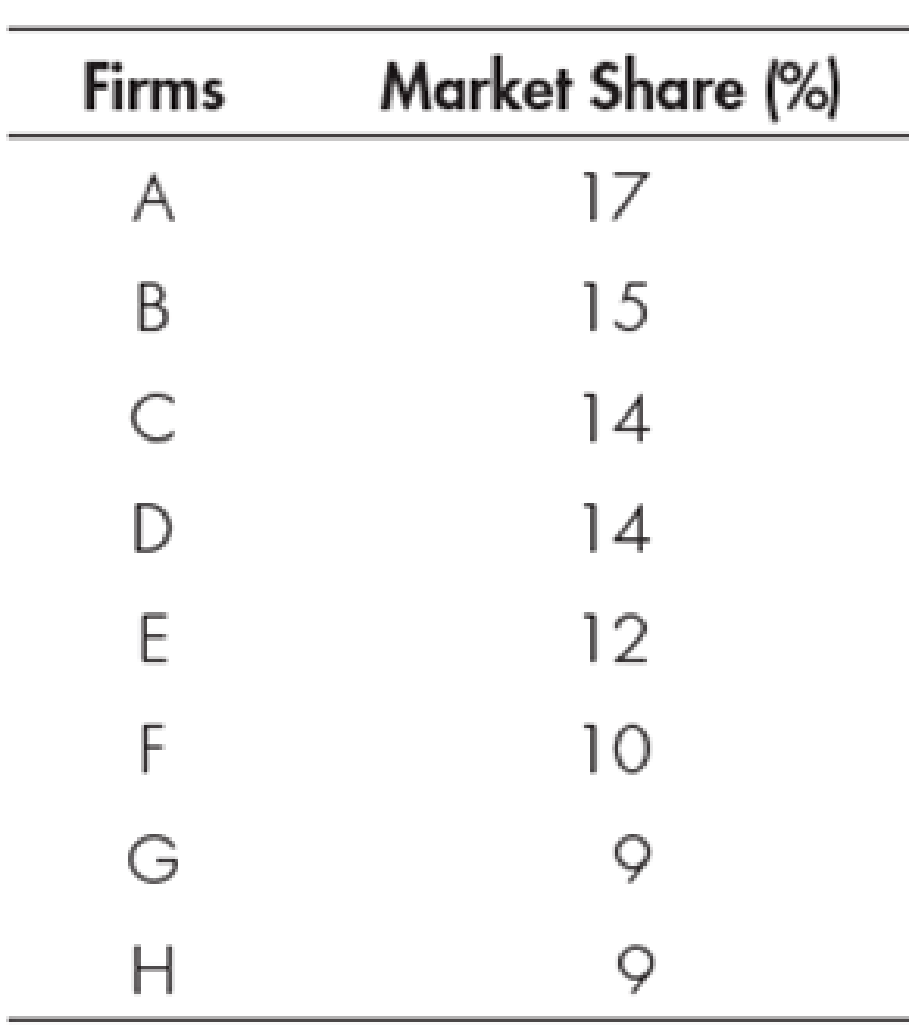Chapter 12, Problem 1WNG

Chapter
Section
Textbook Problem

Calculate the Herfindahl index and the four-firm concentration ratio for the following industry:To determine

Determine Herfindahl index and four-firm concentration ratio.

Explanation

The four-firm concentration ration can be calculated using the formula as follows:

Four-firm concentration ratio=Highest market share of the four firms        (1)

Herfindahl index can be calculated using the formula as follows:

Herfindahl index=(S1)2+(S2)2++(Sn)2        (2)

Here

S1 and Sn are the market shares.

Table-1 shows the value of the market share of the firms as follows:

Use Equation -1 to calculate the four-firm concentration ratio as follows:

Four-firm concentration ratio=17%+15%+14%+14%=60%

Therefore, the four-firm concentration ratio is 60%

Still sussing out bartleby?

Check out a sample textbook solution.

See a sample solution

The Solution to Your Study Problems

Bartleby provides explanations to thousands of textbook problems written by our experts, many with advanced degrees!

Get Started

Why do public utilities generally use different capital structures than biotechnology companies?

Fundamentals of Financial Management, Concise Edition (MindTap Course List)

Assume that you have a short investment horizon (less than 1 year). You are considering two investments: a 1-ye...

Fundamentals of Financial Management, Concise Edition (with Thomson ONE - Business School Edition, 1 term (6 months) Printed Access Card) (MindTap Course List)

Calculate the missing items in the following:

College Accounting (Book Only): A Career Approach